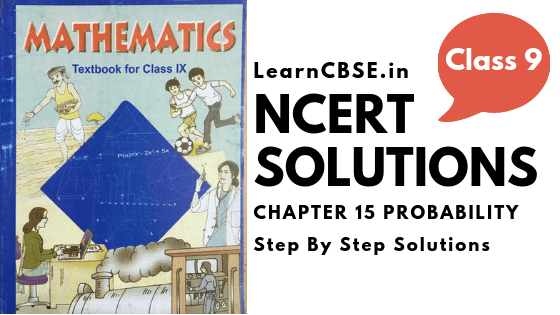NCERT Solutions for Class 9 Maths Chapter 15 Probability Ex 15.1 are part of NCERT Solutions for Class 9 Maths. Here we have given NCERT Solutions for Class 9 Maths Chapter 15 Probability Ex 15.1.

## NCERT Solutions for Class 9 Maths Chapter 15 Probability Ex 15.1

Ex 15.1 Class 9 Maths Question 1.
In a cricket match, a batswoman hits a boundary 6 times out of 30 balls she plays. Find the probability that she did not hit a boundary.
Solution:
Here, the total number of trials = 30
∵ Number of times, the ball touched the boundary =6
∴ Number of times, the ball missed the boundary = 30 – 6 = 24
Let the event of not hitting the boundary be represented by E, then
$$P(E)=\frac { [No.of\quad times\quad the\quad batswoman\quad did\quad not\quad hit\quad the\quad boundary] }{ [Total\quad number\quad of\quad balls\quad she\quad played] } =\quad \frac { 24 }{ 30 } \quad =\quad \frac { 4 }{ 5 }$$
Thus, the required probability = $$\frac { 4 }{ 5 }$$

Ex 15.1 Class 9 Maths Question 2.
1500 families with 2 children were selected randomly, and the following data were recorded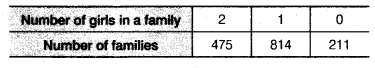Compute the probability of a family, chosen at random, having
(i) 2 girls
(ii) 1 girl
(iii) no girl
Also, check whether the sum of these probabilities is 1.
Solution:
Here, total number of families = 1500

(i) ∵ Number of families having 2 girls = 475
∴ Probability of selecting a family having 2 girls = $$\frac { 475 }{ 1500 } \quad =\quad \frac { 19 }{ 60 }$$

(ii) ∵ Number of families having 1 girl = 814
∴ Probability of selecting a family having 1 girl = $$\frac { 814 }{ 1500 } \quad =\quad \frac { 407 }{ 750 }$$

(iii) Number of families having no girl = 211
Probability of selecting a family having no girl = $$\frac { 211 }{ 1500 }$$
Now, the sum of the obtained probabilities
$$\quad =\frac { 19 }{ 60 } +\frac { 407 }{ 750 } +\frac { 211 }{ 1500 } =\frac { 475+814+211 }{ 1500 } =\frac { 1500 }{ 1500 } =1$$
i.e., Sum of the above probabilities is 1.

Ex 15.1 Class 9 Maths Question 3.
In a particular section of class IX, 40 students were asked about the month of their birth and the following graph was prepared for the data so obtained.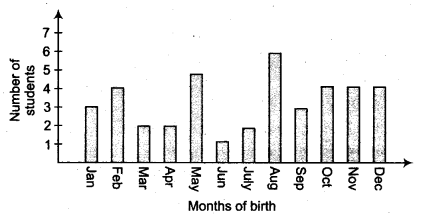Find the probability that a student of the class was born in August.
Solution:
From the graph, we have
Total number of students bom in various months = 40
Number of students bom in August = 6
∴ Probability of a student of the Class-IX who was bom in August $$\quad =\frac { 6 }{ 40 } +\frac { 3 }{ 20 }$$

Ex 15.1 Class 9 Maths Question 4.
Three coins are tossed simultaneously 200 times with the following frequencies of different outcomes.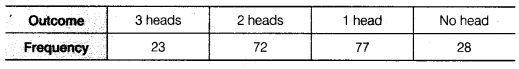If the three coins are simultaneously tossed again, compute the probability of 2 heads coming up.
Solution:
Total number of times the three coins are tossed = 200
Number of outcomes in which 2 heads coming up = 72
∴ Probability of 2 heads coming up = $$\frac { 72 }{ 200 } \quad =\quad \frac { 9 }{ 25 }$$
∴ Thus, the required probability = $$\frac { 9 }{ 25 }$$

Ex 15.1 Class 9 Maths Question 5.
An organisation selected 2400 families at random and surveyed them to determine a relationship between income level and the number of vehicles in a family. The information gathered is listed in the table below.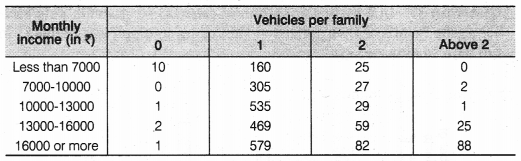Suppose a family is chosen. Find the probability that the family chosen is
(i) earning ₹ 10000-13000 per month and owning exactly 2 vehicles.
(ii) earning ₹16000 or more per month and owning exactly 1 vehicle.
(iii) earning less than ₹ 7000 per month and does not own any vehicle.
(iv) earning ₹13000-16000 per month and owning more than 2 vehicles.
(v) owning not more than 1 vehicle.
Solution:
Here, total number of families = 2400
(i) ∵ Number of families earning Rs. 10000 – Rs. 13000 per month and owning exactly 2 vehicles = 29
∴ Probability of a family earning Rs. 10000 – Rs. 13000 per month and owning exactly 2 vehicles = $$\frac { 29 }{ 2400 }$$

(ii) ∵ Number of families earning Rs. 16000 or more per month and owning exactly 1 vehicle = 579
∴ Probability of a family earning Rs. 16000 or more per month and owning exactly 1 vehicle = $$\frac { 579 }{ 2400 }$$

(iii) ∵ Number of families earning less than Rs. 7000 per month and do not own any vehicle = 10
∴ Probability of a family earning less than Rs. 7000 per month and does not own any vehicle = $$\frac { 10 }{ 2400 }$$ = $$\frac { 1 }{ 240 }$$

(iv) ∵ Number of families earning Rs. 13000 – Rs. 16000 per month and owning more than 2 vehicles = 25
∴ Probability of a family earning Rs. 13000 – Rs. 16000 per month and owning more than 2 vehicles = $$\frac { 25 }{ 2400 }$$ = $$\frac { 1 }{ 96 }$$

(v) ∵ Number of families owning not more than 1 vehicle
= [Number of families having no vehicle] + [Number of families having only 1 vehicle]
= [10 + 1 + 2 + 1] + [160 + 305 + 535 + 469 + 579] = 14 + 2048 = 2062
∴ Probability of a family owning not more than 1 vehicle = $$\frac { 2062 }{ 2400 }$$ = $$\frac { 1031 }{ 1200 }$$

Ex 15.1 Class 9 Maths Question 6.
A teacher wanted to analyse the performance of two sections of students in a mathematics test of 100 marks. Looking at their performances, she found that a few students got under 20 marks and a few got 70 marks or above. So she decided to group them into intervals of varying sizes as follows
0 – 20, 20 – 30, …, 60 – 70, 70 – 100. Then she formed the following table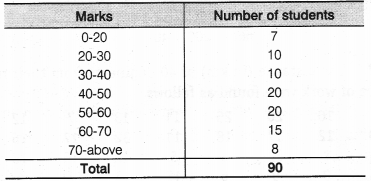(i) Find the probability that a student obtained less than 20% in the mathematics test.
(ii) Find the probability that a student obtained marks 60 or above.
Solution:
Total number of students = 90
(i) From the given table, number of students who obtained less than 20% marks = 7
Probability of a student obtaining less than 20% marks = $$\frac { 7 }{ 90 }$$

(ii) From the given table, number of students who obtained marks 60 or above = [Number of students in class-interval 60 – 70] + [Number of students in the class interval 70 – above]
= 15 + 8 = 23
∴ Probability of a student who obtained 23 marks 60 or above = $$\frac { 23 }{ 90 }$$

Ex 15.1 Class 9 Maths Question 7.
To know the opinion of the students about the subject statistics, a survey of 200 students was conducted. The data is recorded in the following table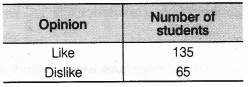Find the probability that a student chosen at random
(i) likes statistics,
(ii) does not like it.
Solution:
Total number of students whose opinion is obtained = 200
(i) ∵ Number of students who like statistics = 135
∴ Probability of selecting a student who likes statistics = $$\frac { 135 }{ 200 }$$ = $$\frac { 27 }{ 40 }$$

(ii) ∵ Number of students who do not like statistics = 65
∴ Probability of selecting a student who does not like statistics = $$\frac { 60 }{ 200 }$$ = $$\frac { 13 }{ 40 }$$

Ex 15.1 Class 9 Maths Question 8.
The distance (in km) of 40 engineers from their residence to their place of work were found as follows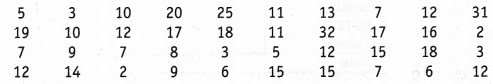What is the empirical probability that an engineer lives
(i) less than 7 km from her place of work?
(ii) more than or equal to 7 km from her place of work?
(iii) within $$\frac { 1 }{ 2 }$$ km from her place of work?
Solution:
Here, total number of engineers = 40

(i) ∵ Number of engineers who are living less than 7 km from their work place = 9
∴ Probability of an engineer who is living less than 7 km from her place of work = $$\frac { 9 }{ 40 }$$

(ii) ∵ Number of engineers living at a distance more than or equal to 7 km from their work place = 31
∴ Probability of an engineer who is living at distance more than or equal to 7 km from her place of work = $$\frac { 31 }{ 40 }$$

(iii) ∵ The number of engineers living within $$\frac { 1 }{ 2 }$$km from their work place = 0
∴ Probability of an engineer who is living within $$\frac { 1 }{ 2 }$$km from her place of work = $$\frac { 0 }{ 40 }$$ = 0

Ex 15.1 Class 9 Maths Question 9.
Activity: Note the frequency of two-wheelers, three-wheelers and four-wheelers going past during a time interval, in front of your school gate. Find the probability that any one vehicle out of the total vehicles you have observed is a two-wheeler?
Solution:
It is an activity. Students can do it themselves.

Ex 15.1 Class 9 Maths Question 10.
Activity : Ask all the students in your class to write a 3-digit number. Choose any student from the room at random. What is the probability that the number written by her/him is divisible by 3? Remember that a number is divisible by 3, if the sum of its digit is divisible by 3.
Solution:
A class room activity for students.

Ex 15.1 Class 9 Maths Question 11.
Eleven bags of wheat flour, each marked 5 kg, actually contained the following weights of flour (in kg)
4.97, 5.05, 5.08, 5.03, 5.00, 5.06, 5.08, 4.98, 5.04, 5.07, 5.00
Find the probability that any of these bags,chosen at random contains more than 5 kg of flour.
Solution:
Here, total number of bags = 11
∵ Number of bags having more than 5 kg of flour = 7
∴ Probability of a bag having more than 5 kg of flour = $$\frac { 7 }{ 11 }$$

Ex 15.1 Class 9 Maths Question 12.
A study was conducted to find out the concentration of sulphur dioxide in the air in parts per million (ppm) of a certain city. The data obtained for 30 days is as followsYou were asked to prepare a frequency distribution table, regarding the concentration of sulphur dioxide in the air in parts per million of a certain city for 30 days. Using this table, find the probability of the concentration of sulphur dioxide in the interval 0.12-0.16 on any of these days.
Solution:
Here, total number of days = 30
∵ The number of days on which the sulphur dioxide concentration is in the interval 0.12 – 0.16 = 2
∴ Probability of a day on which sulphur dioxide is in the interval 0.12 – 0.16 = $$\frac { 2 }{ 30 }$$ = $$\frac { 1 }{ 15 }$$

Ex 15.1 Class 9 Maths Question 13.
The blood groups of 30 students of class VIII are recorded as follows
A, B, 0, 0, AB, 0, A, 0, B, A, 0, B, A, 0, 0, A, AB, 0, A, A, 0, 0, AB, B, A, B, 0
You were asked to prepare a frequency distribution table regarding the blood groups of 30 students of a class. Use this table to determine the probability that a student of this class, selected at random, has blood group AB.
Solution:
Here, total number of students = 30
∵ Number of students having blood group AB = 3
∴ Probability of a student whose blood group is AB = $$\frac { 3 }{ 30 }$$ = $$\frac { 1 }{ 10 }$$.

### NCERT Solutions for Class 9 Maths Chapter 15 Probability (Hindi Medium) Ex 15.1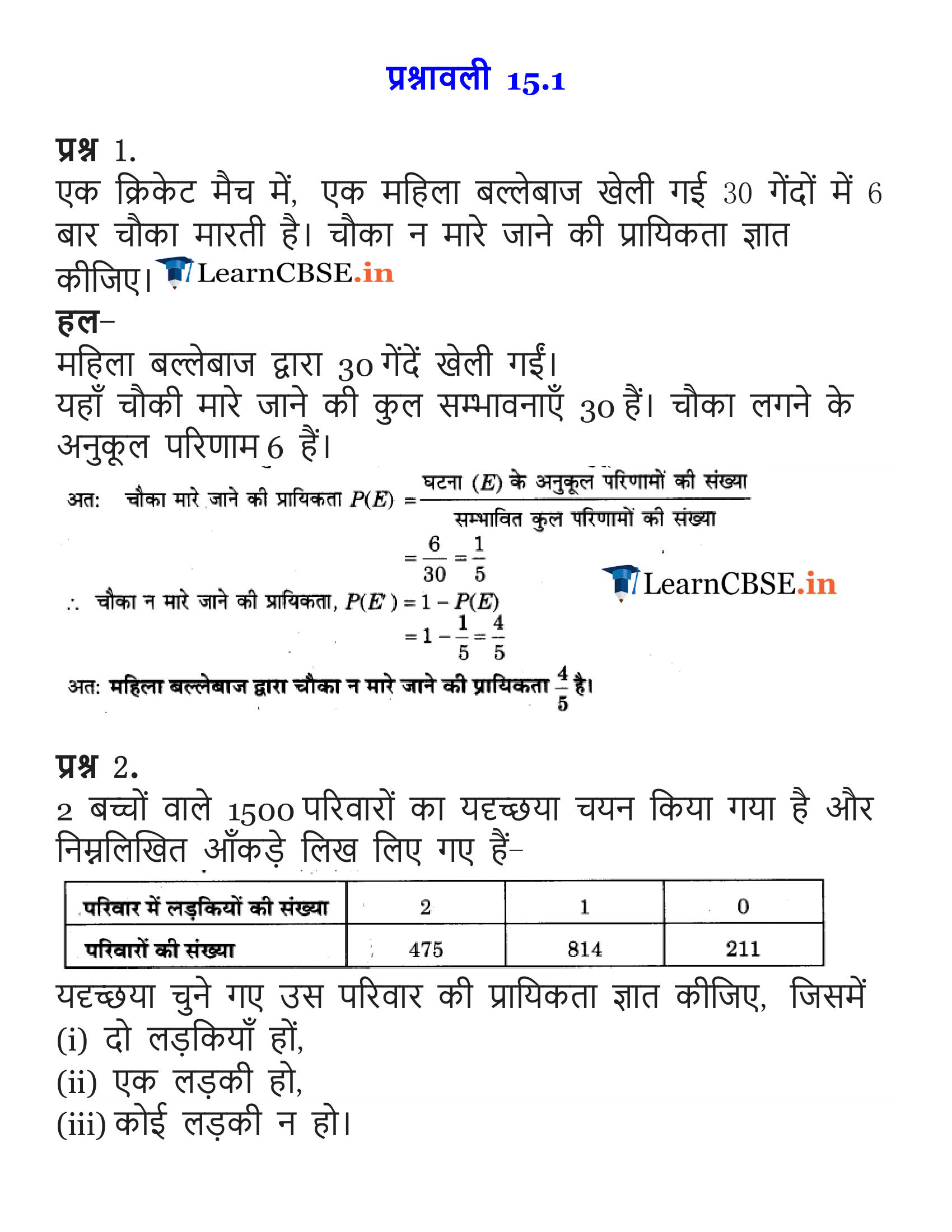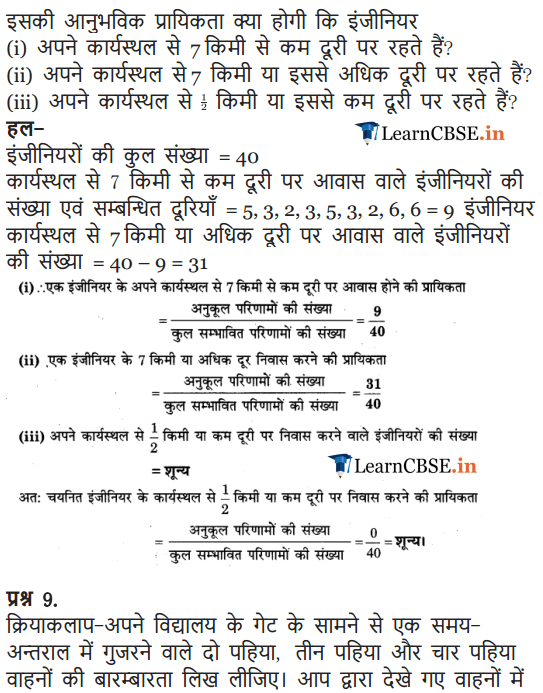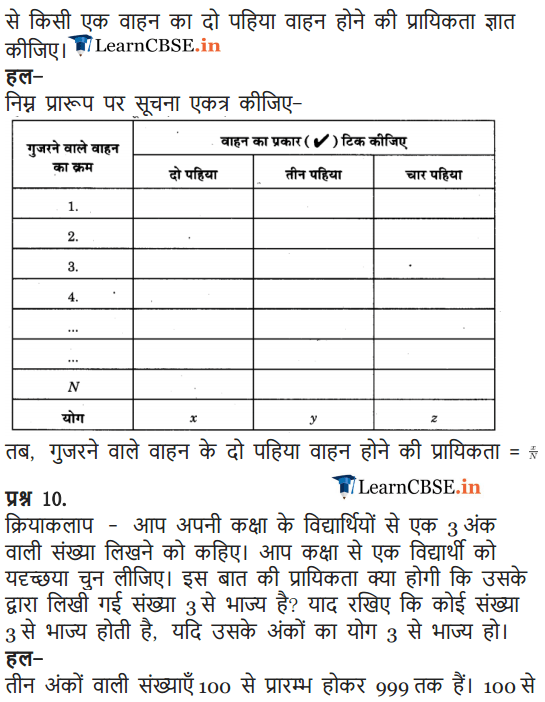We hope the NCERT Solutions for Class 9 Maths Chapter 15 Probability Ex 15.1 help you. If you have any query regarding NCERT Solutions for Class 9 Maths Chapter 15 Probability Ex 15.1, drop a comment below and we will get back to you at the earliest.